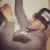Crownnote is where music fans share their favorite songs.

Chart

# Scott's Year-End Chart 3

1. Number
1
2. Number
2
3. Number
3
4. Number
4
5. Number
5
6. Number
6
7. Number
7
8. Number
8
9. Number
9
10. Number
10
11. Number
11
12. Number
12

13. Number
13
14. Number
14
15. Number
15
16. Number
16
17. Number
17
18. Number
18
19. Number
19
20. Number
20
21. Number
21
22. Number
22
23. Number
23
24. Number
24
25. Number
25
26. Number
26
27. Number
27
28. Number
28
29. Number
29

30. Number
30
31. Number
31
32. Number
32
33. Number
33
34. Number
34
35. Number
35
36. Number
36

37. Number
37
38. Number
38

39. Number
39

40. Number
40
41. Number
41

42. Number
42
43. Number
43
44. Number
44
45. Number
45
46. Number
46
47. Number
47

48. Number
48
49. Number
49
50. Number
50
51. Number
51
52. Number
52

53. Number
53
54. Number
54
55. Number
55
56. Number
56
57. Number
57

## New York

58. Number
58
59. Number
59
60. Number
60

A chart byhzeilish
Date
Songs
60
No points